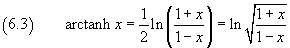## 6. Inverse hyperbolic functions

### arcsinh

The hyperbolic sine is an always increasing function, therefore it is bijective and invertible over all ℜ. If we denote by arcsinh its inverse function, we can write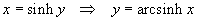From the second one of the (3.9), remembering that ey>0, we have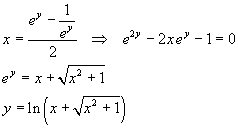and finally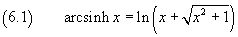### arccosh

The hyperbolic cosine isn't monotonic, so it isn't invertible over all ℜ; to make it invertible we must restrict its range to non negative arguments. Only in this case we can have its inverse function, denoted by arccosh.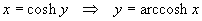From the first one of the (3.9), excluding the negative y,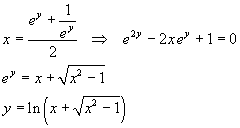and finally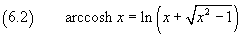### arctanh

The hyperbolic tangent is an always increasing function, therefore it is bijective and invertible over all ℜ. If we denote by arctanh its inverse function, we can write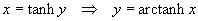From the third one of the (3.9), remembering that e2y>0, we have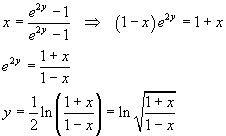and finally